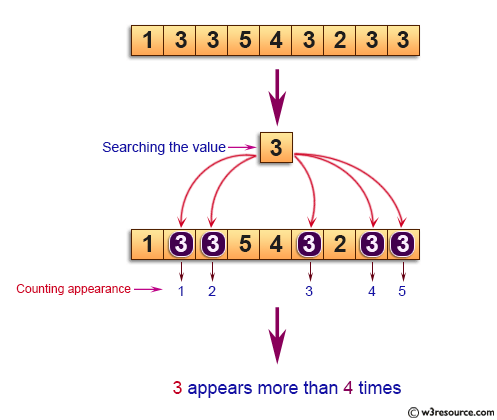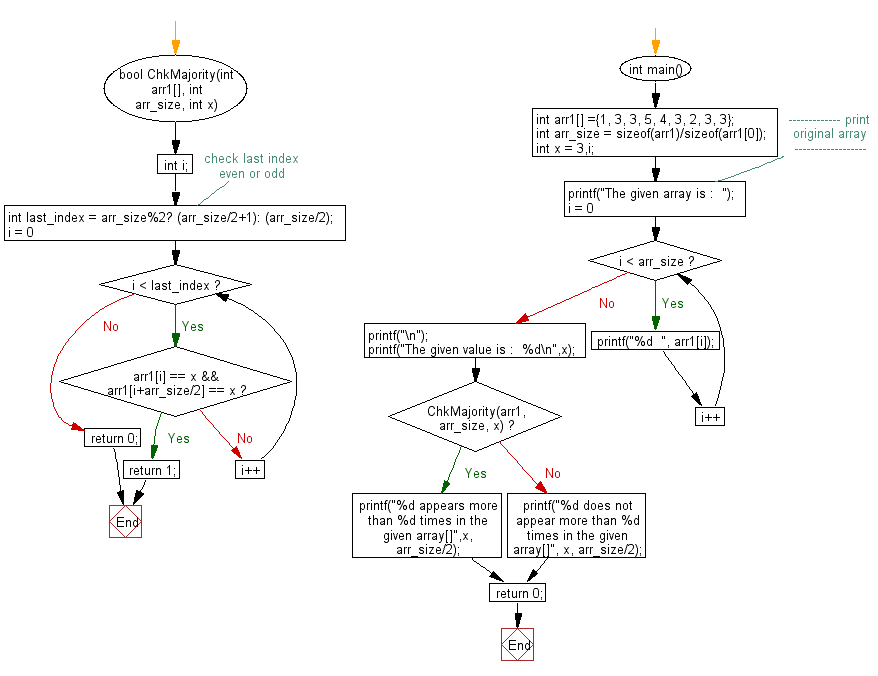﻿ C exercises: Find if a given integer x appears more than n/2 times in a sorted array of n integers - w3resource# C Exercises: Find if a given integer x appears more than n/2 times in a sorted array of n integers

## C Array: Exercise-48 with Solution

Write a program in C to find if a given integer x appears more than n/2 times in a sorted array of n integers.

Pictorial Presentation:Sample Solution:

C Code:

``````# include <stdio.h>
# include <stdbool.h>

bool ChkMajority(int arr1[], int arr_size, int x)
{
int i;
int last_index = arr_size%2? (arr_size/2+1): (arr_size/2);		// check last index even or odd
for (i = 0; i < last_index; i++)
{
if (arr1[i] == x && arr1[i+arr_size/2] == x)
return 1;
}
return 0;
}
int main()
{
int arr1[] ={1, 3, 3, 5, 4, 3, 2, 3, 3};
int arr_size = sizeof(arr1)/sizeof(arr1);
int x = 3,i;
//------------- print original array ------------------
printf("The given array is :  ");
for(i = 0; i < arr_size; i++)
{
printf("%d  ", arr1[i]);
}
printf("\n");
printf("The given value is :  %d\n",x);
//------------------------------------------------------

if (ChkMajority(arr1, arr_size, x))
printf("%d appears more than %d times in the given array[]",x, arr_size/2);
else
printf("%d does not appear more than %d times in the given array[]", x, arr_size/2);

return 0;
}```
```

Sample Output:

```The given array is :  1  3  3  5  4  3  2  3  3
The given value is :  3
3 appears more than 4 times in the given array[]
```

Flowchart:C Programming Code Editor:

Improve this sample solution and post your code through Disqus.

What is the difficulty level of this exercise?

﻿